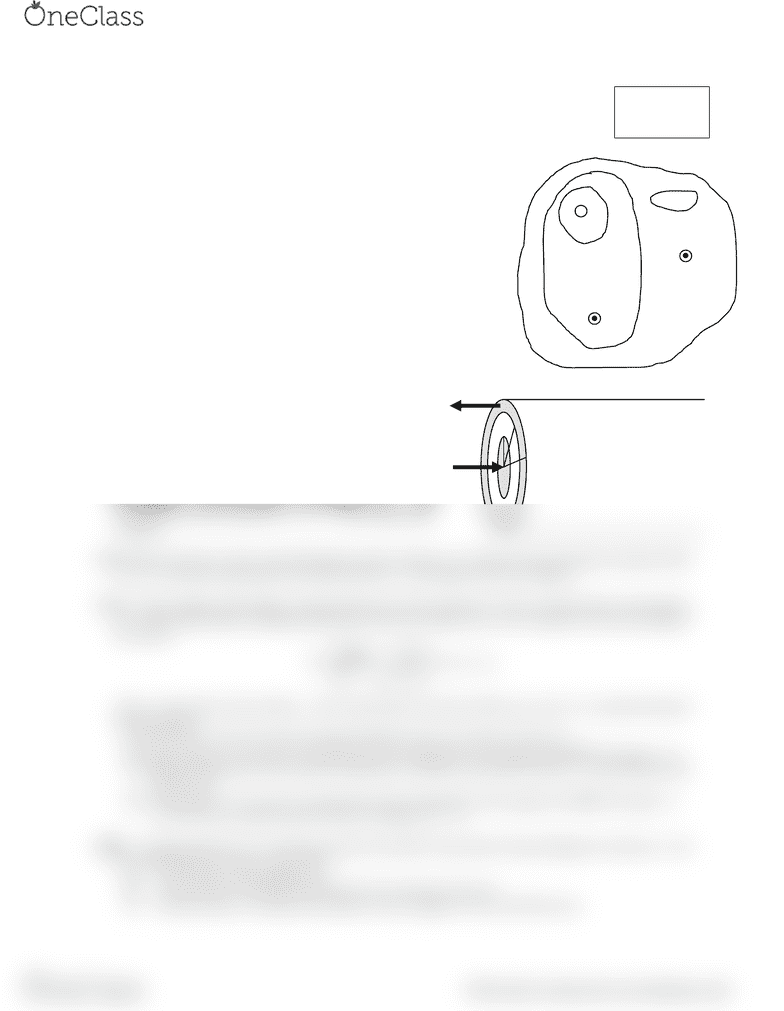Class Notes (1,100,000)
US (460,000)
UC-Irvine (10,000)
MATH (1,000)
MATH 2B (600)
Lecture 15

# MATH 2B Lecture 15: HW20-S15

Department
Mathematics
Course Code
MATH 2B
Professor
Lecture
15

This preview shows half of the first page. to view the full 1 pages of the document.Physics 112 HW20
Due Monday, 13 April 2015
A01. A closed curve encircles several conductors. The line integral
around this curve is 3.83×10-4 T·m.
a) What is the net current in the conductors?
b) If you were to integrate around the curve in the opposite direction,
what would be the value of the line integral? Explain.
A02. The figure at right shows, in cross-section, three conductors that carry
currents through the plane of the figure. The currents have the magnitudes
I1 = 4.0 A, I2 = 6.0 A, I3 = 2.0 A, and the directions shown. Four paths,
labeled a through d, are shown. What is the line integral of
for each
path? Each integral involves going around the path in the counterclockwise
A03. A very long solid cylindrical conductor of radius a carries a
current I to the left as shown at right. Assume this current is
evenly distributed throughout its cross-sectional area.
Concentric with this is a cylindrical shell of inner radius b and
outer radius c. This carries an equal current I in the opposite
direction, evenly distributed throughout the shell. Use
Ampère’s Law to derive expressions for the magnetic fields for
all distances r from the center of the middle wire. Your
expressions will be in terms of a, b, c, r, I, and fundamental
constants.
A04. Repeat A03 above if the current through the central conductor is I1 and the current through the cylindrical shell
is I2 ≠ I1, and both currents point in the same directionto the right in the above diagram.
A05. A long, straight, solid cylinder, oriented with its axis in the z-direction, carries a current whose current density
is J. The current density, although symmetrical about the cylinder axis, is not constant but varies according to
the relation




where a is the radius of the cylinder, r is the radial distance from the cylinder axis, and Io is a constant having the
units of amperes.
a) Show that Io is the total current passing through the entire cross section of the wire.
b) Using Ampère’s Law, derive an expression for the magnitude of the magnetic field
in the region r a.
c) Obtain an expression for the current I contained in a circular cross section of radius r a and centered at the
cylinder axis.
d) Using Ampère’s Law, derive an expression from the magnitude of the magnetic field
in the region r
a. How do your results in parts (b) and (d) compare for r = a?
BI07. A magnetic field of 37.2 T has been achieved at the MIT Francis Bitter National Magnetic Laboratory. Find
the current needed to achieve such a field
a) 2.00 cm from a long straight wire;
b) At the center of a circular coil of radius 42.0 cm that has 100 turns;
c) Near the center of a solenoid with radius 2.40 cm, length 32.0 cm, and 40000 turns.
a
b
c
I
I
×
I1
I2
I3
a
b
c
d
/6
find more resources at oneclass.com
find more resources at oneclass.com